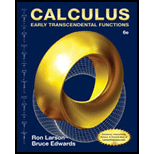Chapter 7.3, Problem 13E### Calculus: Early Transcendental Fun...

6th Edition
Ron Larson + 1 other
ISBN: 9781285774770

#### Solutions

Chapter
Section### Calculus: Early Transcendental Fun...

6th Edition
Ron Larson + 1 other
ISBN: 9781285774770
Textbook Problem

# Finding the Volume of a SolidIn Exercises 1–14, use the shell method to set up and evaluate the integral that gives the volume of the solid generated by revolving the plane region about the y-axis. y = 1 2 π e − x 2 / 2 ,   y = 0 ,   x = 0 ,   x = 1

To determine

To calculate:

The integral y=12πex22 using Shell method that gives the volume of the solid generated by revolving the plain region about y axis.

Explanation

Given:

y=12πex22,y=0,x=0,x=1

Formula used:

Use Shell method Vertical Axis of Revolution.

V=2πabp(x)h(x)dx

Where,

p(x)= Radius of revolution.h(x)= Height of the shell.dx= Thickness of the shell.

Calculation:

We have y=12πex22,y=0,x=0,x=1

Now, apply the Shell method to calculate the volume of solid.

V=2πabp(x)h(x)dx

Where,

p(x)=xh(x)=12πex22

We now have all the expressions in the terms of x.

Since these expressions = the volume of 1 shell,

We must integrate to sum all the shells between x=0 and x=1,

V=2π01x(12πex22)dxV=2π×12<

### Still sussing out bartleby?

Check out a sample textbook solution.

See a sample solution

#### The Solution to Your Study Problems

Bartleby provides explanations to thousands of textbook problems written by our experts, many with advanced degrees!

Get Started

## Additional Math Solutions

#### In Exercises 33-38, rewrite the expression using positive exponents only. 37. 120(s + t)3

Applied Calculus for the Managerial, Life, and Social Sciences: A Brief Approach

#### Simplify the expressions in Exercises 97106. (xy)1/3(yx)2/3

Finite Mathematics and Applied Calculus (MindTap Course List)

#### The arc length of y = 3x + 2 from x = 1 to x = 5 is a) 210 b) 52 c) 102 d) 410

Study Guide for Stewart's Single Variable Calculus: Early Transcendentals, 8th

#### True or False: If , then converge absolutely.

Study Guide for Stewart's Multivariable Calculus, 8th